Test: Mensuration- 1

# Test: Mensuration- 1

Test Description

## 5 Questions MCQ Test Quantitative Aptitude (Quant) | Test: Mensuration- 1

Test: Mensuration- 1 for Banking Exams 2022 is part of Quantitative Aptitude (Quant) preparation. The Test: Mensuration- 1 questions and answers have been prepared according to the Banking Exams exam syllabus.The Test: Mensuration- 1 MCQs are made for Banking Exams 2022 Exam. Find important definitions, questions, notes, meanings, examples, exercises, MCQs and online tests for Test: Mensuration- 1 below.
Solutions of Test: Mensuration- 1 questions in English are available as part of our Quantitative Aptitude (Quant) for Banking Exams & Test: Mensuration- 1 solutions in Hindi for Quantitative Aptitude (Quant) course. Download more important topics, notes, lectures and mock test series for Banking Exams Exam by signing up for free. Attempt Test: Mensuration- 1 | 5 questions in 10 minutes | Mock test for Banking Exams preparation | Free important questions MCQ to study Quantitative Aptitude (Quant) for Banking Exams Exam | Download free PDF with solutions
 1 Crore+ students have signed up on EduRev. Have you?
Test: Mensuration- 1 - Question 1

### Anil grows tomatoes in his backyard which is in the shape of a square. Each tomato takes 1 cm2 in his backyard. This year, he has been able to grow 131 more tomatoes than last year. The shape of the backyard remained a square. How many tomatoes did Anil produce this year?

Detailed Solution for Test: Mensuration- 1 - Question 1

Let the area of backyard be x2 this year and y2 last year.

X2- Y2 = 131
(X+Y) (X-Y) = 131

Now, 131 is a prime number (a unique one too. Check out its properties on Google). Also, always identify the prime number given in a question. Might be helpful in cracking the solution.

⇒ (X+Y) (X-Y) = 131 x 1
⇒ X+Y = 131
⇒ X-Y = 1
⇒ 2X = 132
⇒ X = 66 and Y = 65

∴ Number of tomatoes produced this year = 662 = 4356

Choice (C) is therefore, the correct answer.

Test: Mensuration- 1 - Question 2

### PQRS is a circle and circles are drawn with PO, QO, RO and SO as diameters. Areas A and B are marked. A/B is equal to: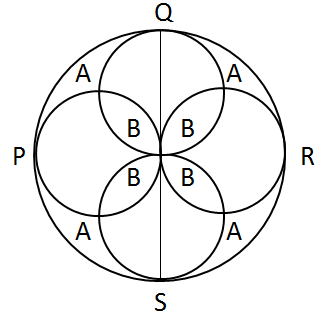Detailed Solution for Test: Mensuration- 1 - Question 2Such questions are all about visualization and ability to write one area in terms of others.

Here, Let the radius of PQRS be 2r
∴ Radius of each of the smaller circles = 2r/2 = r

Area A can be written as:
A = π (2r)2 – 4 x π(r)2 (Area of the four smaller circles) + B (since, B has been counted twice in the previous subtraction)

A = 4πr2 - 4πr2 + B
A = B
A/B = 1

Choice (B) is therefore, the correct answer.

Test: Mensuration- 1 - Question 3

### ABCD is a square drawn inside a square PQRS of sides 4 cm by joining midpoints of the sides PQ, QR, RS, SP. Another square is drawn inside ABCD similarly. This process is repeated infinite number of times. Find the sum of all the squares.

Detailed Solution for Test: Mensuration- 1 - Question 3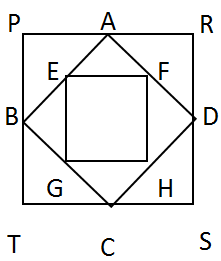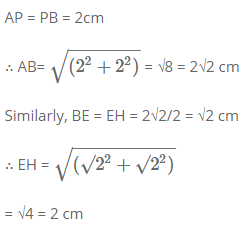If we write the infinite series of area of squares:
= 42 + (2√2)2 + 22 + ……. infinite

Since it is a decreasing series sum of infinite terms can be approximated.
= 16 + 8 + 4 +………infinite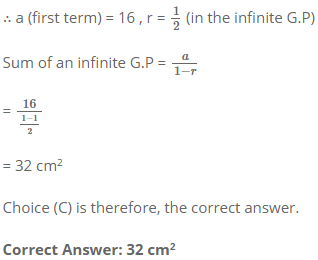Test: Mensuration- 1 - Question 4

PQRST is a pentagon in which all the interior angles are unequal. A circle of radius ‘r’ is inscribed in each of the vertices. Find the area of portion of circles falling inside the pentagon.

Detailed Solution for Test: Mensuration- 1 - Question 4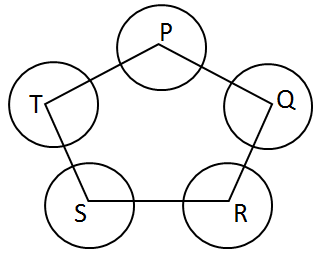Since neither angles nor sides are given in the question, immediately the sum of angles of pentagon should come in mind. To use it,

We know the area of the sectors of a circle is given as: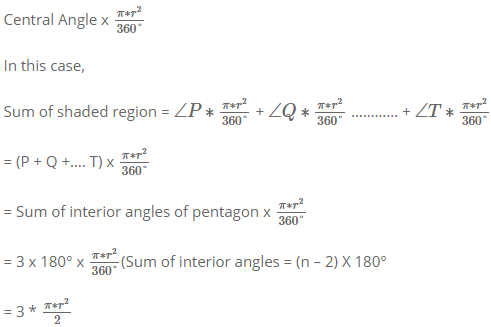Note: The above concept is applicable for a polygon of n sides.

Choice (B) is therefore, the correct answer.

Test: Mensuration- 1 - Question 5

Raj stands in a corner of his square farm. Angle of elevation of a scarecrow placed in diagonally opposite corner is 60°. He starts walking backwards in a straight line and after 80ft he realizes that angle of elevation of the scarecrow now is 30°. What is area of the field?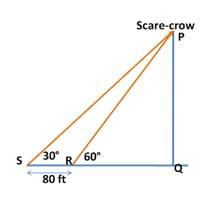Detailed Solution for Test: Mensuration- 1 - Question 5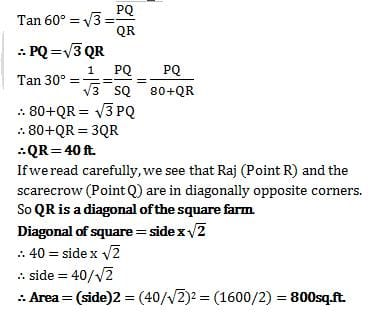## Quantitative Aptitude (Quant)

162 videos|152 docs|131 tests
 Use Code STAYHOME200 and get INR 200 additional OFF Use Coupon Code
Information about Test: Mensuration- 1 Page
In this test you can find the Exam questions for Test: Mensuration- 1 solved & explained in the simplest way possible. Besides giving Questions and answers for Test: Mensuration- 1, EduRev gives you an ample number of Online tests for practice

## Quantitative Aptitude (Quant)

162 videos|152 docs|131 tests